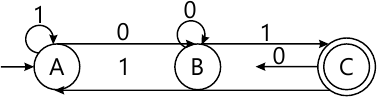MORE IN Theory of Computation
SPPU Computer Engineering (Semester 5)
Theory of Computation
June 2015
Total marks: --
Total time: --
INSTRUCTIONS
(1) Assume appropriate data and state your reasons
(2) Marks are given to the right of every question
(3) Draw neat diagrams wherever necessary

Answer any one question from Q1 and Q2
1 (a) Explain Basic Machines. What are its limitations? How Finite Automata more capable than Basic Machines? Justify with examples.
6 M
1 (b) Write a CFG that generates language L denoted by, (a+b)*.bbb. (a+b)*.
4 M

2 (a) Convert the following finite automation into its equivalent regular expression using Arden's Theorem.6 M
2 (b) If S={a,bb}, find the set of all strings in S* with string length less than or equal to 5. Also for given S, prove whether the following is true or false. (S*)+=(S+)*.
4 M

Answer any one question from Q3 and Q4
3 (a) Design Moore Machine and Mealy Machine to find one's complement of a binary number.
6 M
3 (b) Write the CFG for language L = {0 i 1 j 0 k | j > i +k}. Show the derivation of the string '0111100'.
4 M

4 (a) Define the following and give appropriate examples:
i) Unrestricted Grammar
ii) CFG
iii) Derivation Graph.
6 M
4 (b) Construct FA for the regular expression: (11)*.010. (11)*.
4 M

Answer any one question from Q5 and Q6
5 (a) Design a Turing Machine to recognize an arbitrary string divisible by 4, given ? = {0,1,2}.
10 M
5 (b) Design a Turing Machine that accepts a language L = {0n 1n 0n | n > = 1}
8 M

6 (a) Construct a TM that accepts a language L, a* ba *b.
6 M
6 (b) How can Turing Machines be compared to computers?
6 M
6 (c) Prove that the halting problem in Turing Machines is undecidable.
6 M

Answer any one question from Q7 and Q8
7 (a) Construct transition table for PDA that accepts the language L = {a2n bn | n > =1}. Trace your PDA for the input with n = 3.
10 M
7 (b) Define push down automata (PDA). What are the different types of PDA? Give the applications of PDA.
6 M

8 (a) Give a grammar for the language L(M), where:
$M=(\{q_0, q_1\}, \{0,1\}, \{z_0, x\}, \delta, q_0, z_0, \Phi). \\ And \ \delta \ is \ given \ by: \\ \delta (q_01, z_0) = (q_0\ xz_0) \ \ \ \ \delta (q_0, \varepsilon , z_0)= (q_0, \varepsilon) \\ \delta (q_01, x)= (q_0 \ xx) \ \ \ \ \ \delta (q_r1, x) = (q_1, \varepsilon) \\ \delta (q_00, x)= (q_r,x) \ \ \ \ \ \ \delta (q_00, z_0)=(q_0, z_0)$
8 M
8 (b) Construct PDA for the following regular grammar:
S - > 0A |1B|0
A - > A0|B
B - > c|d
8 M

Answer any one question from Q9 and Q10
9 (a) Justify that the SAT Problem in NP complete.
8 M
9 (b) Explain in detail, the polynomial time reduction approach for proving that a problem is NP Complete.
8 M

10 (a) Explain the Node-Cover Problem with a suitable example.
8 M
10 (b) Explain Tractable and In-tractable Problem.
4 M
10 (c) Justify whether the Travelling Salesman Problem is a class P or class NP problem.
4 M

More question papers from Theory of Computation